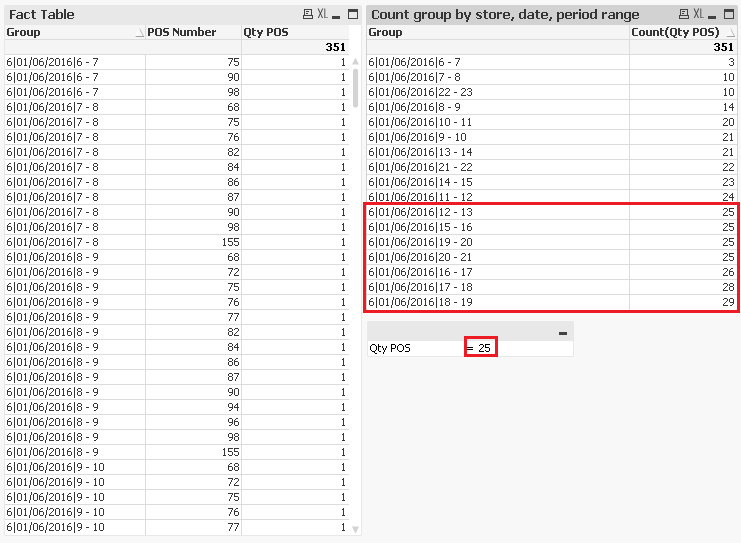# Qlik Sense App Development

Discussion board where members can learn more about Qlik Sense App Development and Usage.Partner

## Calculate peak usage of the POS

Hello,

Can you help me please?

In Table 1, I calculated the maximum use of POS by store, date and period time.

In table 2, shows only the fact table.

I need to know which an expression that returns only the maximum use of the POS as shown in the table 3

Thanks!Tags (1)
9 Replies
Esteemed Contributor II

## Re: Calculate peak usage of the POS

Try as below, or provide a sample app or sample data

=Max(TOTAL <Store>  ExpressionforProductivity )Partner

## Re: Calculate peak usage of the POS

Hi, Vineeth,

the app is attached now.

Esteemed Contributor II

## Re: Calculate peak usage of the POS

Hi,

I'm not able to access my machine that has a licensed QV, can you telll me the fields used / Expressions you have in the table so I can recreatePartner

## Re: Calculate peak usage of the POS

Hi,

Table 1:

Dimensions

Nro_Loja

Qtd_Uso_PDV_FH

Faixa_Horario

Expression

Count(Aggr(if(Rank(Max(Qtd_Uso_PDV_FH),1)=1,1),Nro_Loja,Faixa_Horario))

Table 2: fact table

Dimensions

Nro_Loja

Qtd_Uso_PDV_FH

Expression

Ticket = Sum(Tempo_Cupom)

Availability = Sum(Tempo_Uso)

Productivity = Ticket / Availability

Table 3, is just image. In this table that I need the expression

Esteemed Contributor II

## Re: Calculate peak usage of the POS

Try

Dimension:

Nro_Loja

Expressions:

1) Max(AGGR(MAX(Qtd_Uso_PDV_FH),Nro_Loja))

2) AGGR(if(Qtd_Uso_PDV_FH = MAX(AGGR(NODISTINCT MAX(Qtd_Uso_PDV_FH),Nro_Loja)), sum(Tempo_Cupom)),Nro_Loja,Qtd_Uso_PDV_FH)

3) AGGR(if(Qtd_Uso_PDV_FH = MAX(AGGR(NODISTINCT MAX(Qtd_Uso_PDV_FH),Nro_Loja)), sum(Tempo_Uso)),Nro_Loja,Qtd_Uso_PDV_FH)

4) Colum(2)/Colum(3)Partner

## Re: Calculate peak usage of the POS

Hi,

It worked!!!

Thank you so much!

Esteemed Contributor II

## Re: Calculate peak usage of the POS

Hi claudio

Attaching something simpler

Dimensions:

Nro_Loja

calculatedDimension:

=aggr(if(Qtd_Uso_PDV_FH = Max(TOTAL <Nro_Loja> AGGR(MAX(Qtd_Uso_PDV_FH),Nro_Loja,Qtd_Uso_PDV_FH)) , Qtd_Uso_PDV_FH),Nro_Loja,Qtd_Uso_PDV_FH)

<<Check Suppress when value is null() for this dimension

Expressions:

sum(Tempo_Cupom)

sum(Tempo_Uso)

Column(1)/Column(2)

Also please close the thread by marking a response as correctQlik Community Tip: Marking Replies as Correct or HelpfulPartner

## Re: Calculate peak usage of the POS

Hi,

I have another situation, can you help me, please?

1. Create a bar chart that represents only the time bands where the amount of POS usage is greater than or equal to the value of the variable by calculating the average availability time.

2. Insert a line in the bar chart showing witch is the frequency in periods ranges the amount of POS in use are greater than or equal to the value of the variable.